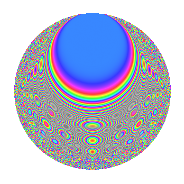# Properties

 Label 403.2.baLevel 403 Weight 2 Character orbit ba Rep. character $$\chi_{403}(6,\cdot)$$ Character field $$\Q(\zeta_{12})$$ Dimension 140 Newforms 1 Sturm bound 74 Trace bound 0

# Related objects

## Defining parameters

 Level: $$N$$ = $$403 = 13 \cdot 31$$ Weight: $$k$$ = $$2$$ Character orbit: $$[\chi]$$ = 403.ba (of order $$12$$ and degree $$4$$) Character conductor: $$\operatorname{cond}(\chi)$$ = $$403$$ Character field: $$\Q(\zeta_{12})$$ Newforms: $$1$$ Sturm bound: $$74$$ Trace bound: $$0$$

## Dimensions

The following table gives the dimensions of various subspaces of $$M_{2}(403, [\chi])$$.

Total New Old
Modular forms 156 156 0
Cusp forms 140 140 0
Eisenstein series 16 16 0

## Trace form

 $$140q - 8q^{2} - 12q^{4} - 2q^{5} + 6q^{6} + 12q^{7} - 10q^{8} - 124q^{9} + O(q^{10})$$ $$140q - 8q^{2} - 12q^{4} - 2q^{5} + 6q^{6} + 12q^{7} - 10q^{8} - 124q^{9} - 6q^{10} - 12q^{11} + 26q^{12} - 6q^{13} - 24q^{14} + 18q^{15} + 48q^{16} - 4q^{18} + 10q^{19} - 50q^{20} - 28q^{21} - 12q^{24} + 6q^{26} - 54q^{28} - 28q^{31} - 10q^{32} - 30q^{33} + 72q^{34} - 8q^{35} + 48q^{36} + 8q^{37} + 72q^{38} - 8q^{39} - 12q^{40} - 20q^{41} + 30q^{42} + 26q^{43} + 24q^{46} + 12q^{47} + 54q^{48} - 108q^{49} + 10q^{50} + 36q^{51} + 46q^{52} + 24q^{53} - 18q^{54} + 24q^{56} - 52q^{57} - 42q^{58} - 10q^{59} - 18q^{60} + 36q^{61} + 12q^{62} - 58q^{63} - 84q^{65} + 16q^{66} + 36q^{67} - 12q^{69} + 30q^{70} + 106q^{71} + 62q^{72} + 20q^{73} - 90q^{74} - 82q^{75} + 20q^{76} - 48q^{77} - 6q^{78} - 48q^{79} + 32q^{80} + 132q^{81} - 6q^{83} - 86q^{84} + 42q^{85} + 6q^{86} - 14q^{87} + 24q^{88} + 36q^{89} - 90q^{90} + 46q^{91} - 58q^{93} + 4q^{94} + 48q^{95} - 54q^{96} + 26q^{97} - 40q^{98} - 18q^{99} + O(q^{100})$$

## Decomposition of $$S_{2}^{\mathrm{new}}(403, [\chi])$$ into irreducible Hecke orbits

Label Dim. $$A$$ Field CM Traces $q$-expansion
$$a_2$$ $$a_3$$ $$a_5$$ $$a_7$$
403.2.ba.a $$140$$ $$3.218$$ None $$-8$$ $$0$$ $$-2$$ $$12$$